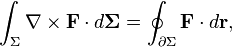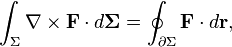Is it possible to derive Divergence Theorem from Stokes Theorem?

Is it? If so, can you show how? ThanksLast edited:

It is possible, what have you attempted?

It is possible, what have you attempted?

I don't know where to start, unfortunately the mathematics disciplines in physics, in my university, are a bit superficial, with no theoretical exercises (only exercises to apply the theory). So I'm not trained for this, I want to see someone doing it so I can learndextercioby
Homework Helper
Well, if by <Stokes Theorem> you mean the Stokes theorem from the theory of differential forms and by <Divergence Theorem> you mean the Gauß-Ostrogradski theorem in integral form, then the answer is <YES>, and the proof of this can be found in several places, such as any book with advanced mathematical methods for physicists (Arfken-Weber, Szekeres, Nakahara,...).

lavinia
Gold Member
I don't know where to start, unfortunately the mathematics disciplines in physics, in my university, are a bit superficial, with no theoretical exercises (only exercises to apply the theory). So I'm not trained for this, I want to see someone doing it so I can learnin three space there is a natural duality between vector fields and 2 forms.

I'm still not sure if the OP is talking about the general Stokes theorem with differential forms or the vector calculus Stokes theorem relating the circulation of a vector field to the surface integral of the curl. I'm having a hard time seeing how to derive the divergence theorem from the latter, but I can derive the Stokes circulation theorem from the divergence theorem pretty easily (with the help of the identity div (A x B) = B . curl A - A . curl B ).

I'm wondering what deluks917 had in mind...

I'm referring to the Kelvin-Stokes theoremI thought so. I haven't figured out how to get the divergence theorem from that theorem. I'm looking forward to seeing how deluks917 does it. It would seem that you have to slice the volume into thin slices and then use the Kelvin-Stokes theorem (on each slice) and some vector identities to arrive at the divergence theorem, but I keep hitting a snag along the way. In the meantime maybe you could try deriving the Kelvin-Stokes theorem from the divergence theorem. It is pretty much the reverse of the process I just described. Expand the portion of a surface S slightly along its normal vector field into a thin shell volume V of thickness h << 1. That way you can apply the divergence theorem to a certain vector field. The vector identity

div (A x B) = B . curl A - A . curl B

is useful. Also, on the volume V you have curl N = 0, where N is the normal vector field from S extended along itself a small distance h. If you can do that, then maybe you will see what I've been missing and do the derivation in the other direction, too. Note that what I'm talking about is a very physics-book style "proof"... it would make mathematicians giggle.

Tosh5457, any luck deriving Kelvin-Stokes from the Divergence theorem or vice-versa?

lavinia
Gold Member
I'm referring to the Kelvin-Stokes theoremThe Divergence theorem does not refer to line integrals. However the generalized Stokes theorem implies the Divergence Theorem.

the duality I referred to above is (a,b,c) -> ady^dz - bdx^dz + cdx^dy

The exterior derivative of this 2 form is the divergence of the vector field (a,b,c) times the Euclidean volume element. Have fun figuring out the rest.

Last edited:
If you apply the divergence theorem to the vector field FxN over the thin-shell volume (thickness h<<1) that I described above, you can get the Kelvin-Stokes theorem. S is a portion of a surface bounded by a closed curve C. N is the normal vector field from the surface portion S extended to the volume V. So on the top and bottom surface of the shell, N coincides with n, the outward unit normal to surface that bounds V. Along the edge of the shell, however, the outward normal is given by n = t x N, where t is the unit tangent to C.

$$\int_{\partial V} (\mathbf{F} \times \mathbf{N})\cdot \mathbf{n} dS = \int_{V} \nabla \cdot (\mathbf{F} \times \mathbf{N}) dV = \int_{V} \mathbf{N} \cdot (\nabla \times \mathbf{F}) dV = h\int_{S} \mathbf{N} \cdot (\nabla \times \mathbf{F}) dS + o(h)$$

Also,

$$\int_{\partial V} (\mathbf{F} \times \mathbf{N})\cdot \mathbf{n} dS = \int_{\mbox{top surface}} (\mathbf{F} \times \mathbf{N})\cdot \mathbf{N} dS + \int_{\mbox{bottom surface}} (\mathbf{F} \times \mathbf{N})\cdot \mathbf{N} dS + \int_{\mbox{edge}} (\mathbf{F} \times \mathbf{N})\cdot (\mathbf{t} \times \mathbf{N}) dS$$

The first two integrals on the right hand side are obviously zero. The last one is

$$\int_{\mbox{edge}} (\mathbf{F} \times \mathbf{N})\cdot (\mathbf{t} \times \mathbf{N}) dS = \int_{\mbox{edge}} \mathbf{F}\cdot \mathbf{t} dS = h\int_{C} \mathbf{F}\cdot \mathbf{t} dl + o(h)$$

I wish I could see how to do it in the other direction.